# Class 8 Maths NCERT Solutions for Chapter – 3 Understanding Quadrilaterals Ex – 3.3

Question 1.
Given a parallelogram ABCD. Complete each statement along with the definition with the definiton or property used.

(i)
(ii) ∠DCB = …………….
(iii) OC = ……………….
(iv) m∠DAB + m∠CDA = …………..

Solution.
(i) AD = BC : In a parallelogram, opposite sides are equal.
(ii) ∠DCB = ∠DAB : In a parallelogram, opposite angles are equal.
(iii) OC = OA : The diagonals of a parallelogram bisect each other.
(iv) m∠DAB + m∠CDA = 180° : In a parallelogram, the stun of any two adjacent angles is 180°.

Question 2.
Consider the following parallelograms. Find the values of the unknowns x, y, z.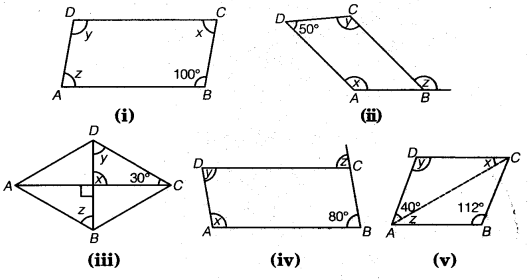Solution.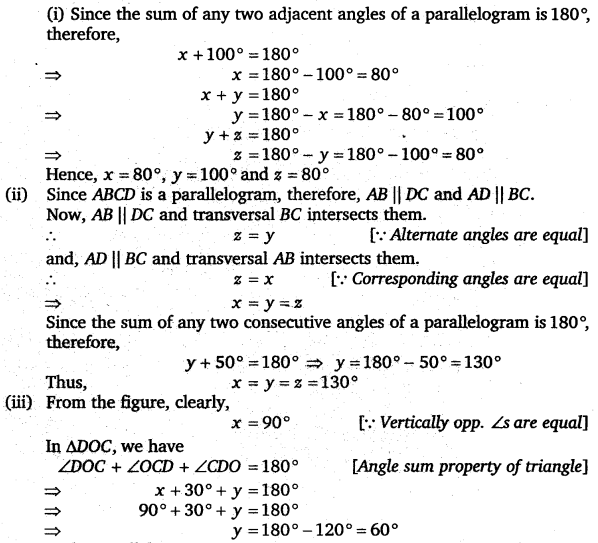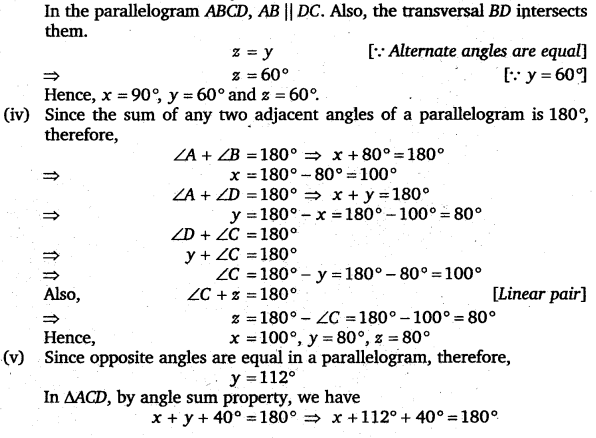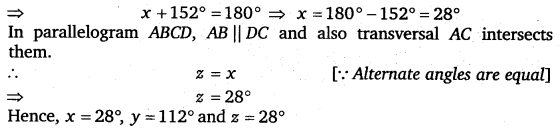Question 3.
Can a quadrilateral ABCD be a parallelogram if
(i) ∠D + ∠B = 180° ?
(ii) AB = DC = 8 cm, AD = 4 cm and BC = 4.4 cm
(iii) ∠A = 70° and ∠C = 65°?

Solution.
(i) If in a quadrilateral ABCD, ∠D + ∠B = 180°, then it is not necessary that ABCD is a parallelogram.
(ii) Since AD ≠ BC, i.e., the opposite sides are unequal, so ABCD is not a parallelogram.
(iii) Since ∠A ≠ ∠C, i.e., the opposite angles are unequal, so ABCD is not a parallelogram.

Question 4.
Draw a rough figure of a quadrilateral that is not a parallelogram but has exactly two opposite angles of equal measure.

Solution.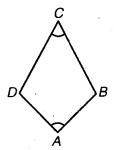A rough figure of a quadrilateral ABCD that is not a parallelogram has been drawn with exactly two opposite angles of equal measure such that ∠A – ∠C which is a kite as an example.

Question 5.
The measures of two adjacent angles of a parallelogram are in the ratio 3 :2. Find the measure of each of the angles of the parallelogram.

Solution.
Let two adjacent angles A and B of ||gm ABCD be 3x and 2x respectively.
Since the adjacent angles of a parallelogram are supplementary.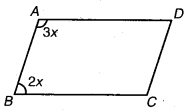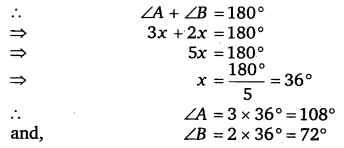Since the opposite angles are equal in a parallelogram, therefore,
∠C = ∠A = 108° and ∠D = ∠B = 72°
Hence, ∠A = 108°, ∠B = 72°, ∠C = 108° and ∠D = 72°.

Question 6.
Two adjacent angles of a parallelogram have equal measure. Find the measure of each of the angles of the parallelogram.

Solution.
Let two adjacent angles A and B of parallelogram ABCD be x each.
Since the adjacent angles of a parallelogram are supplementary.Question 7.
The adjacent figure HOPE is a parallelogram. Find the angle measures x, y and z. State the properties you use to find them.

Solution.

Since HOPE is a parallelogram, therefore, HE || OP and HO || EP.
Now, HE || OP and transversal HO intersects them.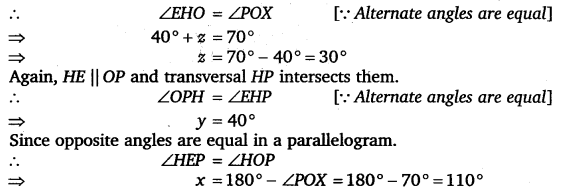Hence, x = 110°, y = 40° and z = 30°

Question 8.
The following figures GUNS and RUNS are parallelograms. Find x and y. (Lengths are in cm)

Solution.
(i) Since GUNS is a parallelogram, therefore, its opposite sides are equal.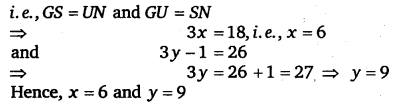(ii) In a parallelogram, diagonals bisect each other, therefore,Question 9.In the below figure both RISK and CLUE are parallelograms. Find the value of x.

Solution.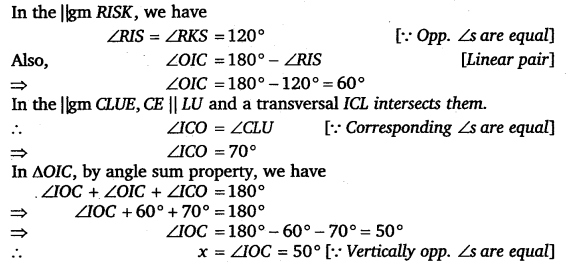Question 10.
Explain how this figure is a trapezium. Which of its two sides are parallel ?

Solution.

Since ∠KLM + ∠NML = 180° i.e., the pair of consecutive interior angles are supplmentary.
Therefore, KL || NM and so KLMN is a trapezium.

Question 11.
Find m∠C in the figure, if$\overline { AB }$ ||$\overline { DC }$.

Solution.

Since AB || DC and transversal BC intersects
them.
∠B + ∠C= 180°
[∵ Sum of interior angles is 180°]
⇒ 120°+ ∠C =180° A
⇒ ∠C = 180°- 120° = 60°
Hence, m∠C =60°

Question 12.
Find the measure of ∠P and ∠S, if$\overline { SP }$ ||$\overline { RQ }$ in the figure. (If you find mZ R, is there more than one method to find m∠P ?)

Solution.

Since SP || RQ and PQ is a transversal intersecting them at P and Q.
∴ ∠P + ∠Q =180°
[∵ Sum of interior angles is 180°]